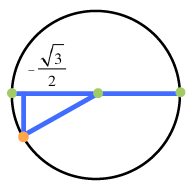### Home > PC3 > Chapter 3 > Lesson 3.2.1 > Problem3-98

3-98.

Determine the exact value of each of the following trigonometric expressions without using a calculator.1. $\cos\left(\frac{7\pi}{6}\right)$

Draw a unit circle with reference angle.1. $\sin\left(\frac{3\pi}{2}\right)$

Follow the hint in part (a).

1. $\tan\left(\frac{3\pi}{2}\right)$

What is the slope of the line associated with this angle?

1. $\tan\left(\frac{2\pi}{3}\right)$

Follow the hint in part (a).